The UCM Procedure

FORECAST Statement

• FORECAST <options>;

The FORECAST statement is an optional statement that is used to specify the overall forecasting environment for the specified model. It can be used to specify the span of observations, the historical period, to use to compute the forecasts of the future observations. This is done using the SKIPFIRST= and BACK= options. The number of periods to forecast beyond the historical period, and the significance level of the forecast confidence interval, is specified using the LEAD= and ALPHA= options. You can request one-step-ahead series and component forecasts by using the PRINT= option. You can save the series forecasts, and the model-based decomposition of the series, in a data set by using the OUTFOR= option. You can use the BOOTSTRAP option to request the computation of bootstrap prediction standard errors and the associated confidence intervals. The following example illustrates the use of this statement:

```   forecast skipfirst=12 back=24 lead=30;
```

This statement requests that the initial 12 and the last 24 response values be excluded during the forecast computations. The forecast horizon, specified using the LEAD= option, is 30 periods; that is, multistep forecasting begins at the end of the historical period and continues for 30 periods. The actual observation span used to compute the multistep forecasting is decided as follows: Suppose that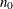and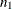are the observation numbers of the first and the last nonmissing values of the response variable, respectively. As a result of SKIPFIRST=12 and BACK=24, the historical period, or the forecast span, begins at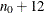and ends at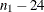. Multistep forecasts are produced for the next 30 periods—that is, for the observation numbers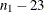to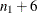. Of course, the forecast computations can fail if the model has regressor variables that have missing values in the forecast span. If the regressors contain missing values in the forecast horizon—that is, between the observationsand—the forecast horizon is reduced accordingly.

ALPHA=value

specifies the significance level of the forecast confidence intervals; for example, ALPHA=0.05, which is the default, results in a 95% confidence interval.

BACK=integer
SKIPLAST=integer

specifies the holdout sample for the evaluation of the forecasting performance of the model. For example, BACK=10 results in treating the last 10 observed values of the response series as unobserved. A post-sample-prediction-analysis table is produced for comparing the predicted values with the actual values in the holdout period. The default is BACK=0.

BOOTSTRAP(NREP=integer <SEED=integer>)

enables the computation of bootstrap prediction standard errors based on the specified number of replications (NREP). The value of NREP must be at least 2. Optionally, you can specify the random number seed that is associated with the first replication by using the SEED= option. The seeds for the subsequent replications are assigned sequentially. The default seed value that is associated with the first replication is 123. The BOOTSTRAP option has no effect if the number of parameters to be estimated is zero (that is, all the model parameters are known). Note that this option is computationally expensive. The computational cost of NREP replications is comparable to the cost of estimating parameters NREP times.

enables continuation of the diffuse filtering iterations for k additional iterations beyond the first instance where the initialization of the diffuse state would have otherwise taken place. If the specified k is larger than the sample size, the diffuse iterations continue until the end of the sample. Note that one-step-ahead forecasts are produced only after the diffuse state is initialized. Delaying the initialization leads to reduction in the number of one-step-ahead forecasts. This option is useful when you want to ignore the first few one-step-ahead forecasts that often have large variance.

specifies the number of periods to forecast beyond the historical period defined by the SKIPFIRST= and BACK= options; for example, LEAD=10 results in the forecasting of 10 future values of the response series. The default is LEAD=12.

OUTFOR=SAS-data-set

specifies an output data set for the forecasts. The output data set contains the ID variable (if specified), the response and predictor series, the one-step-ahead and out-of-sample response series forecasts, the forecast confidence intervals, the smoothed values of the response series, and the smoothed forecasts produced as a result of the model-based decomposition of the series.

PLOT=DECOMP
PLOT=DECOMPVAR
PLOT=FDECOMP
PLOT=FDECOMPVAR
PLOT=FORECASTS
PLOT=TREND
PLOT=( <plot request> …<plot request> )

requests forecast and model decomposition plots. The FORECASTS option provides the plot of the series forecasts, the TREND and DECOMP options provide the plots of the smoothed trend and other decompositions, the DECOMPVAR option can be used to plot the variance of these components, and the FDECOMP and FDECOMPVAR options provide the same plots for the filtered decomposition estimates and their variances.

PRINT=DECOMP
PRINT=FDECOMP
PRINT=FORECASTS
PRINT=NONE
PRINT=( <print request> …<print request> )

controls the printing of the series forecasts and the printing of smoothed model decomposition estimates. By default, the series forecasts are printed only for the forecast horizon specified by the LEAD= option; that is, the one-step-ahead predicted values are not printed. You can request forecasts for the entire forecast span by specifying the PRINT=FORECASTS option. Using PRINT=DECOMP, you can get smoothed estimates of the following effects: trend, trend plus regression, trend plus regression plus cycle, and sum of all components except the irregular. If some of these effects are absent in the model, then they are ignored. Similarly you can get filtered estimates of these effects by using PRINT=FDECOMP. You can use PRINT=NONE to suppress the printing of all the forecast output.

SKIPFIRST=integer

indicates that some early part of the data needs to be ignored during the forecasting calculations. This can be useful if there is a reason to believe that the model being used for forecasting is not appropriate for this portion of the data. SKIPFIRST=10 results in skipping the first 10 measurements of the response series during the forecast calculations. The default is SKIPFIRST=0.